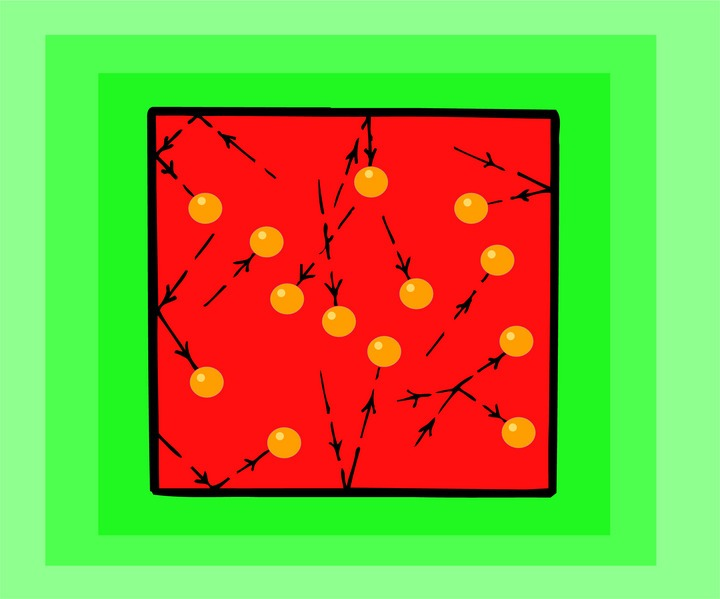# Day 95

## Kinetic Theory

The Kinetic Theory is the cornerstone of all of knowledge and assumptions about matter. There are two key points:

1. All matter is made up of particles that are in constant motion.

2. Collisions between these particles are perfectly elastic.

The motion of the particles depends on the average kinetic energy of the particles, the measurement of which is called temperature. So the temperature of a substance determines how fast the particles move, which also determines how often they collide.

There are forces of attraction between the particles of a substance. The closer the particles are, the stronger the forces, and vice versa.

As seen in a previous unit, this is what determines the phase in which substances exist.

The ‘exception’ is gases, although not so much an exception as an extension. The particles of gases are so far apart that, for all practical purposes, there are no viable forces holding them together.

There are three laws of thermodynamics that, together with the Kinetic Theory (often called the fourth law), describe all phase changes and temperature changes of substances.## Ideal Gas Law

An ideal gas is a theoretical gas consisting of infinitely small molecules that exert no forces on each other. It consists of a collection of atoms or molecules that move randomly, exert no long-range forces on one another, and occupy a negligible fraction of the volume of their container. Although actual gases are not ideal, in most cases, their behavior is similar enough to an ideal gas to allow us to use the Ideal Gas Law to explain and predict their behaviors.

The laws that explain and define the behavior of gases are:

Charles Law states that the volume of a dry gas is directly proportional to its Kelvin temperature, provided the pressure remains constant;

when pressure is held constant

V/T is a constant for any gas

When comparing samples of gas,

V1/T1 = V2/T2

Go to CSUDH. You can click on New Problem and get several examples to try. Be sure you check your answers.

At Software Systems website there is a Charles Law calculator to try.

Boyles Law states that the volume of a dry gas varies inversely with the pressure exerted on it, provided the temperature remains constant;

when temperature is constant,

PV = constant

When comparing samples of a gas,

P1V1 = P2V2

These laws were combined into the Combined Gas Equation which relates the temperature, pressure and volume of any gas.

P1V1/T1 = P2V2/T2

(NOTE: The temperatures in the above laws must be stated in Kelvin.)

Finally, the Italian physicist, Amedeo Avogadro experimented and postulated about the molecular weight of a gas and the number of molecules in one gram-molecular weight (mole) of any substance. Avogadros number is the number of molecules in one gram-molecular weight of a substance and it is equal to

6.02 X 1023

The value of PV/T in the combined gas equation is designated by the symbol R and is the same for one mole of any gas. This value is independent of the chemical composition of the gas (except at very high pressures) and is known as the universal gas constant. For any given sample of an ideal gas:

PV = nRT

Therefore when pressure is expressed in Pa and volume in m3, R has the value of

R = 8.31 J/mol-K

Although this defines the behavior of the ideal gas, it does describe the behavior of real gases with reasonable accuracy except at low temperatures, extreme pressures, or both.

(NOTE: Refresher one mole (mol) of any substance is that amount of the substance that contains as many particles as there are atoms in 12 g of the carbon-12 isotope. This number is Avogadros number.)

(source)